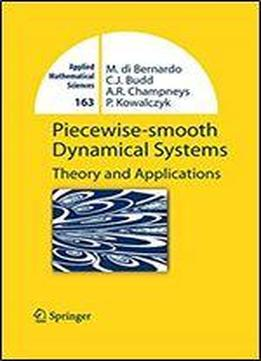# Piecewise-smooth Dynamical Systems: Theory And Applications (applied Mathematical Sciences Book 163) by Mario Bernardo / 2008 / English / PDF

This book presents a coherent framework for understanding the dynamics of piecewise-smooth and hybrid systems. An informal introduction expounds the ubiquity of such models via numerous. The results are presented in an informal style, and illustrated with many examples. The book is aimed at a wide audience of applied mathematicians, engineers and scientists at the beginning postgraduate level. Almost no mathematical background is assumed other than basic calculus and algebra.

views: 350